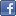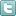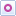EE Times-India > Controls/MCUs

Controls/MCUs# Reducing the cost of field-oriented motor control

Posted: 04 May 2015Print Version

Keywords:Field-oriented control  FOC  motor control  motor shaft sensor  sensorless

This may not be intuitive at first, but consider this. If we take any closed-loop system utilising negative feedback, we can drive the error signal to nearly zero if we have high enough loop gain. In this case, an error of zero means the phase current estimate is equal to the measured value of phase current. But this can only be true if the signal at the back-EMF input actually equals the missing back-EMF voltage (assuming Ls and Rs estimates are accurate). In other words, the PI controller's high gain servos the error signal to zero by forcing the controller's output to become the back-EMF signal.

Figure 5 shows a Spice simulation of a single-phase back-EMF observer running at 10kHz. The back-EMF signal inside of the motor block is unknown to the observer. But once the simulation starts, the observer output quickly converges on the back-EMF signal as shown in figure 6. Even when you change the back-EMF waveform to different wave shapes, the observer morphs its output to match it!Figure 5: Spice model of back-EMF observer.Figure 6: Simulation output showing observer performance.

From figure 4, we can create the back-EMF waveforms for the alpha and beta coils, but just having the back-EMF signals doesn't mean we are done. The angle information is still embedded in these sinusoidal waveforms, and we have to figure out how to extract it. One way is to use the arc tan function:Besides requiring floating point capability to calculate this value, you will also need to trap for the singularity when EMFalpha(t) = 0. But consider another technique (borrowed from the world of resolvers) shown in figure 7. Not only does it solve the problems associated with the arc tan function, it offers another very nice perk: a filtered speed signal! A free Spice simulation of an entire three-phase back-EMF sensorless algorithm based on figure 7 can be downloaded here.Figure 7: Angle demodulator using the relationship: sin(θ)cos(θest)-cos(θ)sin(θest) = sin(θ-θest).

Now that we have solved for the angle, I need to tell you that we solved for the wrong angle. What we wanted was the angle of the rotor flux vector. What we get is the angle of the back-EMF vector.

Before you close your browser in disgust, you should also know that the back-EMF vector always lies on the quadrature axis, so we are very close! All that remains is to determine the polarity of the back-EMF vector on the q-axis in order to know exactly where the rotor flux vector is. This is where the frequency signal from figure 7 can help. If the frequency is positive, we subtract 90 degrees from the back-EMF angle. If it is negative, we add 90 degrees instead. Now that we have the angle of the rotor flux, we can proceed with the four steps of FOC presented in my previous series.

Wow! All these calculations, just to find the angle of the rotor flux vector! But considering the low cost of embedded MIPS compared to the high cost of a motor shaft sensor, it is still way worth the effort in most cases.

In my next article, I will conclude this series by introducing a new sensorless FOC algorithm, which is available in ROM on select TI C2000 microcontroller products. If you are new to sensorless motor control, or are just tired of writing your own sensorless algorithm every time, then you will definitely want to stay tuned for my next article!

Dave Wilson is Senior Industrial Systems Engineer for C2000 Microcontrollers at Texas Instruments.

 Related Articles Editor's Choice
Comment on "Reducing the cost of field-oriented ..."

Top Ranked Articles

WebinarsVisit Asia Webinars to learn about the latest in technology and get practical design tips.

Search EE Times India
Services

Go to topConnect on FacebookFollow us on TwitterFollow us on Orkut
﻿